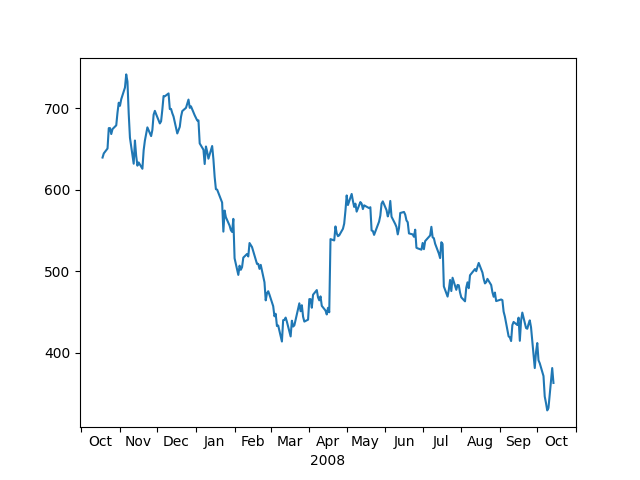# Centering labels between ticks¶

Ticklabels are aligned relative to their associated tick. The alignment 'center', 'left', or 'right' can be controlled using the horizontal alignment property:

for label in ax.xaxis.get_xticklabels():
label.set_horizontalalignment('right')


However there is no direct way to center the labels between ticks. To fake this behavior, one can place a label on the minor ticks in between the major ticks, and hide the major tick labels and minor ticks.

Here is an example that labels the months, centered between the ticks.import numpy as np
import matplotlib.cbook as cbook
import matplotlib.dates as dates
import matplotlib.ticker as ticker
import matplotlib.pyplot as plt

# load some financial data; Google's stock price
r = (cbook.get_sample_data('goog.npz', np_load=True)['price_data']
.view(np.recarray))
r = r[-250:]  # get the last 250 days

fig, ax = plt.subplots()

ax.xaxis.set_major_locator(dates.MonthLocator())
# 16 is a slight approximation since months differ in number of days.
ax.xaxis.set_minor_locator(dates.MonthLocator(bymonthday=16))

ax.xaxis.set_major_formatter(ticker.NullFormatter())
ax.xaxis.set_minor_formatter(dates.DateFormatter('%b'))

for tick in ax.xaxis.get_minor_ticks():
tick.tick1line.set_markersize(0)
tick.tick2line.set_markersize(0)
tick.label1.set_horizontalalignment('center')

imid = len(r) // 2
ax.set_xlabel(str(r.date[imid].item().year))
plt.show()


Keywords: matplotlib code example, codex, python plot, pyplot Gallery generated by Sphinx-Gallery